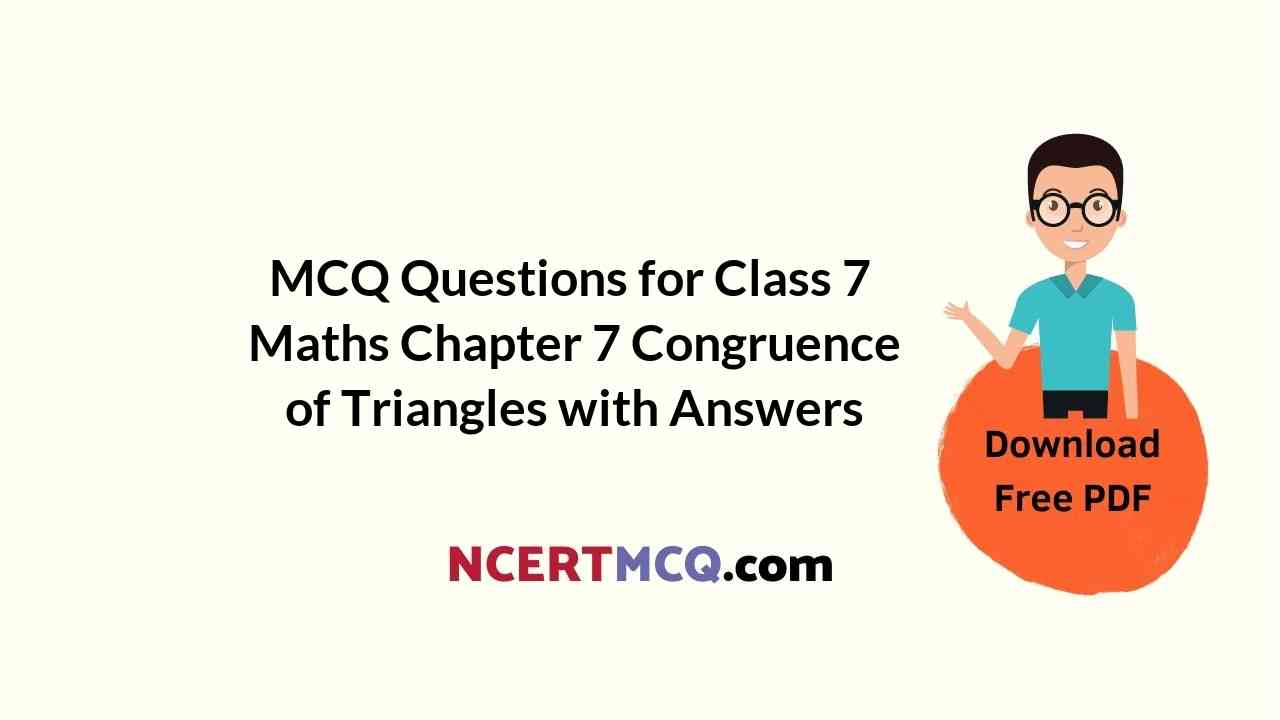Check the below Online Education NCERT MCQ Questions for Class 7 Maths Chapter 7 Congruence of Triangles with Answers Pdf free download. MCQ Questions for Class 7 Maths with Answers were prepared based on the latest exam pattern. We have provided Congruence of Triangles Class 7 Maths MCQs Questions with Answers to help students understand the concept very well. https://ncertmcq.com/mcq-questions-for-class-7-maths-with-answers/

Students can also refer to NCERT Solutions for Class 7 Maths Chapter 7 Congruence of Triangles for better exam preparation and score more marks.

## Online Education for Congruence of Triangles Class 7 MCQs Questions with Answers

Congruence Of Triangles Class 7 MCQ Question 1.
‘Under a given correspondence, two triangles are congruent if the three sides of the one are equal to the three corresponding sides of the other.’
The above is known as
(a) SSS congruence of two triangles
(b) SAS congruence of two triangles
(c) ASA congruence of two triangles
(d) RHS congruence of two right-angled triangles

Answer: (a) SSS congruence of two triangles

Class 7 Maths Chapter 7 MCQ Question 2.
‘Under a given correspondence, two triangles are congruent if two sides and the angle included between them in one of the triangles are equal to the corresponding sides and the angle included between them of the other triangle.’
The above is known as
(a) SSS congruence of two triangles
(b) SAS congruence of two triangles
(c) ASA congruence of two triangles
(d) RHS congruence of two right-angled triangles

Answer: (b) SAS congruence of two triangles

MCQ On Congruence Of Triangles Class 7 Question 3.
‘Under a given correspondence, two triangles are congruent if two angles and the side included between them in one of the triangles are equal to the corresponding angles and the side included between them of the other triangle.’
The above is known as
(а) SSS congruence of two triangles
(b) SAS congruence of two triangles
(c) ASA congruence of two triangles
(d) RHS congruence of two right-angled triangles

Answer: (c) ASA congruence of two triangles

MCQ Questions For Class 7 Maths Chapter 7 Question 4.
‘Under a given correspondence, two right-angled triangles are congruent if the hypotenuse and a leg of one of the triangles are equal to the hypotenuse and the corresponding leg of the other triangle.’
The above is known as
(а) SSS congruence of two triangles
(b) SAS congruence of two triangles
(c) ASA congruence of two triangles
(d) RHS congruence of two right-angled triangles

Answer: (d) RHS congruence of two right-angled triangles

MCQ Questions On Congruence Of Triangles Class 7 Question 5.
For two given triangles ABC and PQR, how many matchings are possible?
(a) 2
(b) 4
(c) 6
(d) 3

Hint:
ABC ↔ PQR, ABC ↔ PRQ,
ABC ↔ QRP, ABC ↔ QPR,
ABC ↔ RPQ, ABC ↔ RQP.

Class 7 Maths Ch 7 MCQ Question 6.
The symbol for congruence is
(a) ≡
(b) ≅
(c) ↔
(d) =

Class 7 Math Chapter 7 MCQ Question 7.
The symbol for correspondence is
(a) =
(b) ↔
(c) ≡
(d) ≅

Class 7 Maths Chapter 7 MCQ With Answers Question 8.
If ∆ ABC = ∆ PQR, then $$\overline { AB }$$ corresponds to
(a) $$\overline { PQ }$$
(b) $$\overline { QR }$$
(c) $$\overline { RP }$$
(d) none of these

Answer: (a) $$\overline { PQ }$$

MCQ Of Congruence Of Triangles Class 7 Question 9.
If ∆ ABC = ∆ PQR, then $$\overline { BC }$$ corresponds to
(a) $$\overline { PQ }$$
(b) $$\overline { QR }$$
(c) $$\overline { RP }$$
(d) none of these

Answer: (b) $$\overline { QR }$$

Class 7th Maths Chapter 7 MCQ Question 10.
If ∆ ABC = ∆ PQR, then $$\overline { CA }$$ corresponds to
(a) $$\overline { PQ }$$
(b) $$\overline { QR }$$
(c) $$\overline { RP }$$
(d) none of these

Answer: (c) $$\overline { RP }$$

Class 7 Chapter 7 Maths MCQ Question 11.
If ∆ ABC = ∆ PQR, then ∠A corresponds to
(a) ∠P
(b) ∠Q
(c) ∠R
(d) none of these

Ncert Class 7 Maths Chapter 7 MCQ Question 12.
If ∆ ABC = ∆ PQR, then ∠B corresponds to
(a) ∠P
(b) ∠Q
(c) ∠R
(d) none of these

Class 7 Congruence Of Triangles MCQ Question 13.
If ∆ ABC= ∆ PQR, then ∠C corresponds to
(a) ∠P
(b) ∠Q
(c) ∠R
(d) none of these

MCQ Questions For Class 7 Maths Congruence Of Triangles Question 14.
We want to show that ∆ ART = ∆ PEN and we have to use SSS criterion. We have AR = PE and RT = EN. What more we need to show?
(a) AT = PN
(b) AT = PE
(c) AT = EN
(d) none of these

Class 7 Congruence Of Triangles Extra Questions Question 15.
We want to show that ∆ ART = ∆ PEN. We have to use SAS criterion. We have ∠T = ∠N, RT = EN. What more we need to show?
(a) PN = AT
(b) PN = AR
(c) PN = RT
(d) None of these

MCQ Class 7 Maths Chapter 7 Question 16.
We want to show that ∆ ART = ∆ PEN. We have to use ASA criterion. We have AT = PN, ∠A = ∠P. What more we need to show?
(a) ∠T = ∠N
(b) ∠T = ∠E
(c) ∠T = ∠P
(d) None of these

Question 17.
Which congruence criterion do you use in the following?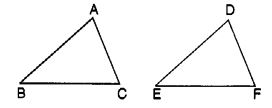Given AC = DF
AB = DE
BC = EF
So, ∆ ABC ≅ ∆ DEF
(a) SSS
(b) SAS
(c) ASA
(d) RHS

Question 18.
Which congruence criterion do you use in the following?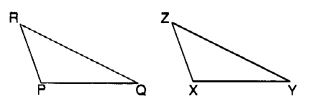Given : ZX = RP
RQ = ZY
∠ PRQ = ∠ XZY
So, ∆ PRQ = ∆ XYZ
(a) SSS
(b) SAS
(c) ASA
(d) RHS

Question 19.
Which congruence criterion do you use in the following?(a) SSS
(b) SAS
(c) ASA
(d) RHS

Question 20.
Which congruence criterion do you use in the following?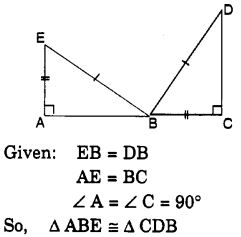(a) SSS
(b) SAS
(c) ASA
(d) RHS

Question 21.
In the following figure, the two triangles are congruent. The corresponding parts are marked. We can write ∆ RAT = ?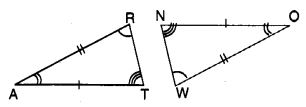(a) ∆ WON
(b) ∆ WNO
(c) ∆ OWN
(d) ∆ ONW

Question 22.
Complete the congruence statement ∆ BCA = ?(a) ∆ BTA
(b) ∆ BAT
(c) ∆ ABT
(d) ∆ ATB

Question 23.
Complete the congruence statement ∆ QRS(a) ∆ TPQ
(b) ∆ TQP
(c) ∆ QTP
(d) ∆ QPT

Question 24.
If ∆ ABC and ∆ PQR are to be congruent, name one additional pair of corresponding parts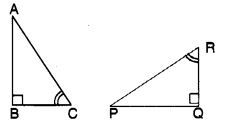(a) BC = QR
(b) BC = PQ
(c) BC = PR
(d) none of these

Question 25.
By which congruence, is ∆ ABC = ∆ FED?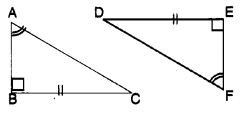(a) SSS
(b) SAS
(c) ASA
(d) RHS